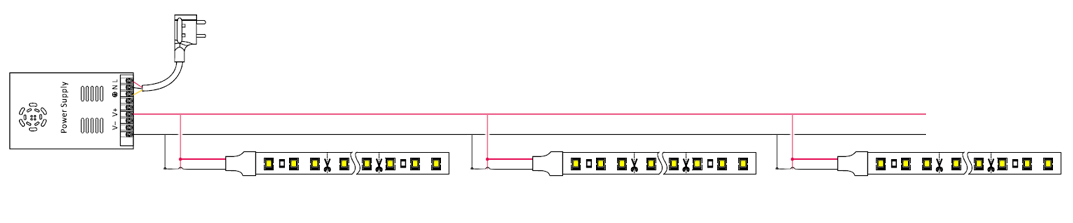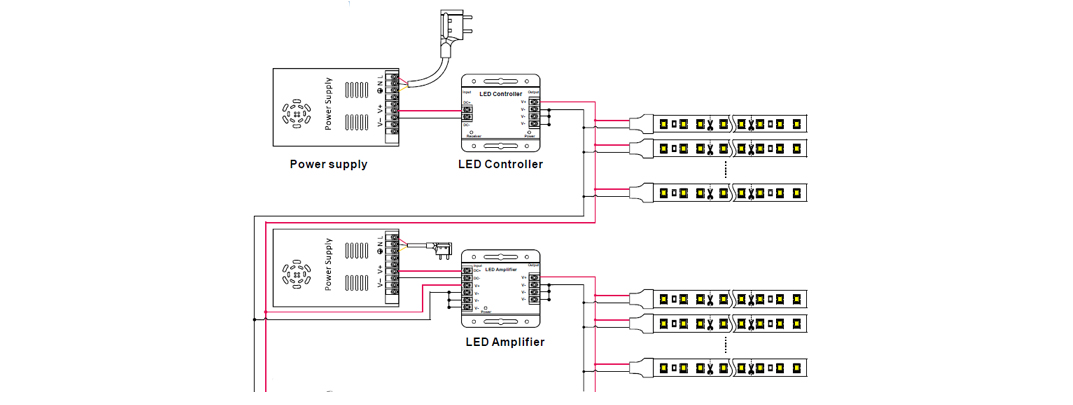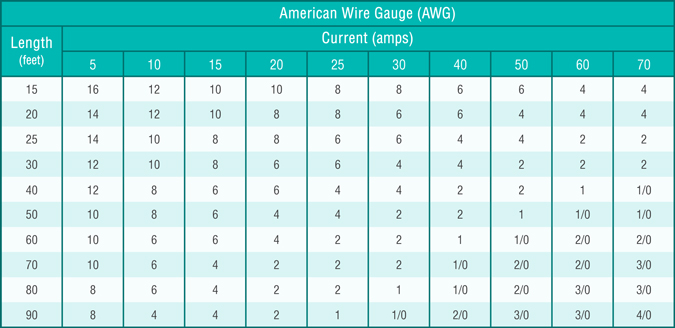Shenzhen Ledodm Lighting Co., Ltd

LED GUIDE

» » » What is voltage drop?

# What is voltage drop?

"Voltage Drop" is one consideration of the most important things when installing led strip lights. In DC circuits, voltage gradually drops as it goes through a length of wire or led strip. So, with every meter of wire or led light strip, the available volatge to each meter gradually decreses along the length, which will lead to brighter one end of your strip lights than the other end.

How to avoid Voltage Drop in LED strip lighting?

Voltage drop will vary based on the material quality, voltage input and power comsumption of the led strip light. 12V led strips will lose more volatge than 24V, we'd like to recommend 24V led strip if you want to make it longer over 5meters. That's why most of our led strips are 5meters per roll. In order to prevent voltage drop, you can divide the long LED strip lights into shorter ones, then connect additional “Parallel” wires from the power supply to each new LED strip light. This process can be repeated as many times as needed when voltage drop starts to occur. Just make sure you are using the correct wire gauge as explained below.

Example A: Running multiple parallel runs at longer distancesExample B: Create a parallel connection from the power supply and split the runs in different directionsExample C: Use seperate power supplies in different areas(controller optional for single color, and it's a must if rgb color)How to determine the correct Wire Gauge?

1. Calculate the total length of wire from the power supply to the LED strip.

2. Determine the amount of current (amps) in the wire. To do this, add the total length of LED strips that are connected to the power supply, and multiply by the wattage per meter of the led strip.

Example: SMD3528 60LEDs/m consumes 4.8 watts per meter. If you are using 5meters, (5 x 4.8) = 24 watts

3. Divide the total wattage of your strips by 12 (if using 12 volt strips) to get the total current (amps). If you are using 24 volt strips, divide by 24. Now you have the current (amps).

Example 1: 24 watts divided by 12 = 2 amps

Example 2: 24 watts divided by 24 = 1 amps

4. The correct wire gauge is found in the intersection of amps and feet as seen in the chart below.Note: The wire itself draws current. If you are running longer lengths of wire than the total length of strips, use the next thickest wire. On the AWG scale, a LOWER number indicates a THICKER wire.

It’s never a good idea to continuously run any electronic circuit at maximum capacity. For best results, add an additional 20% margin to your calculations. For example, if your total power consumption is 24 watts, add 20% (watts x 1.2) and assume it’s 28.8 watts. Then continue to perform your calculations for power supplies and wire gauges. This will protect your system to make it last longer and operate more safely.

Subscribe to our newsletters. Free Sample Available!